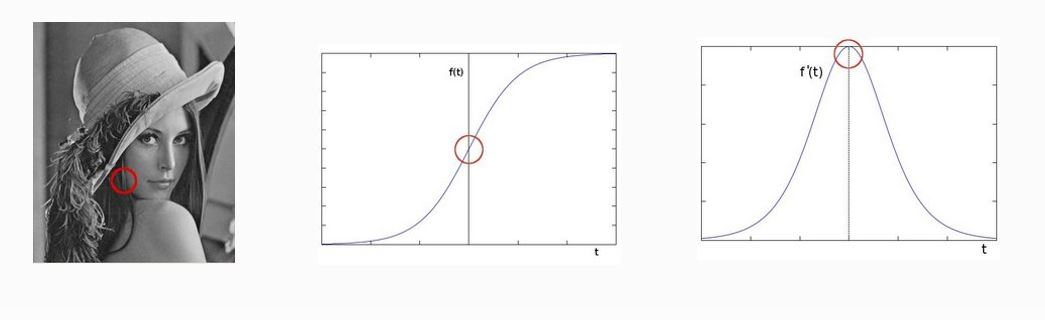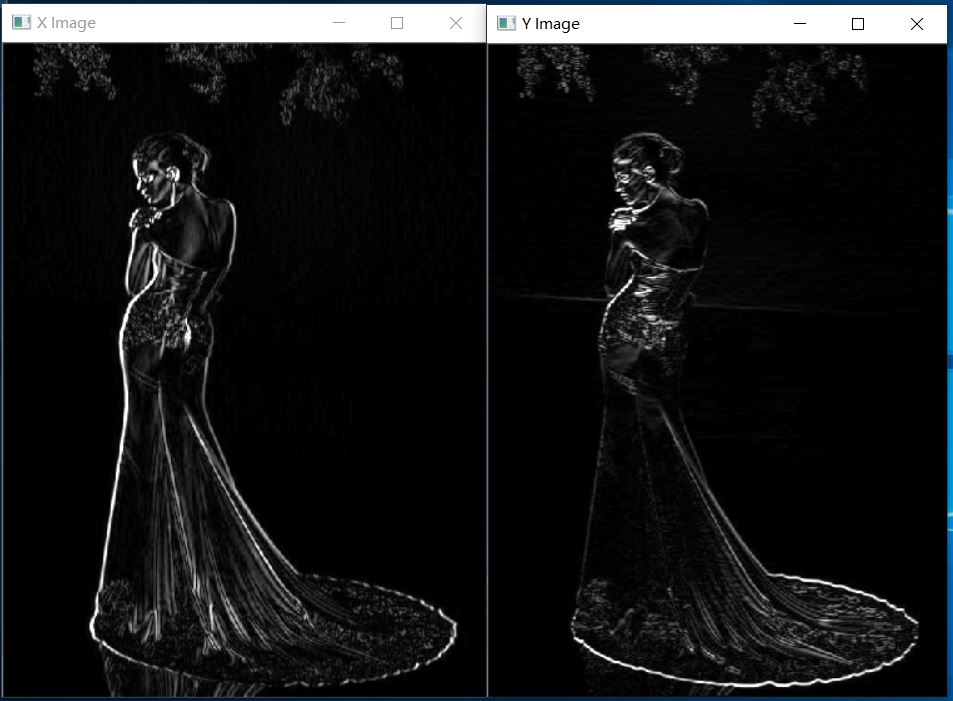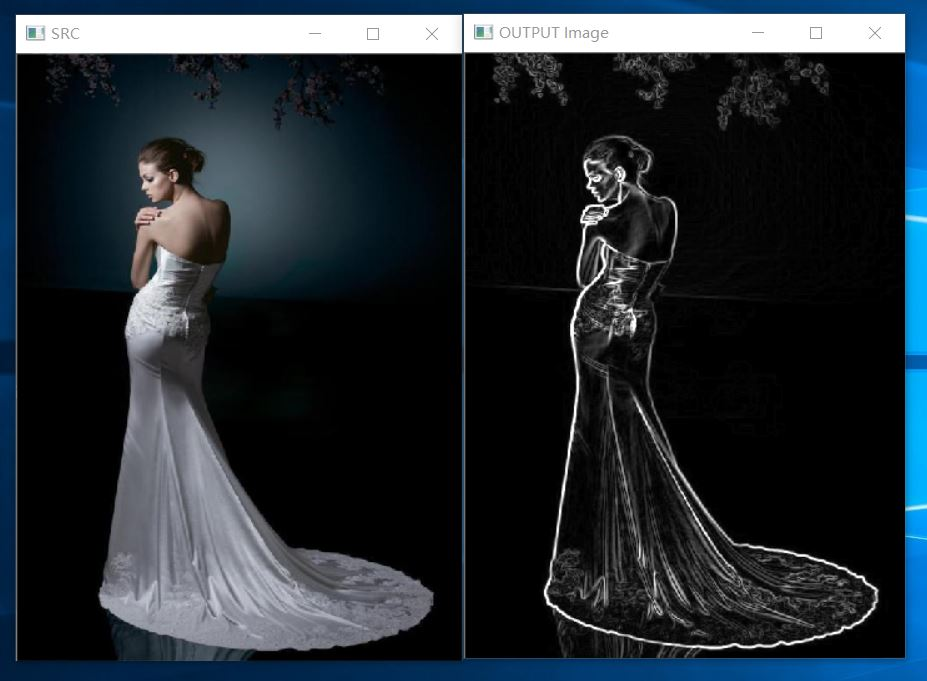2019-06-09 20:55:09 weixin_41049188 阅读数 659
• ###### MATLAB图像处理

全面系统的学习MATLAB在图像处理中的应用

20781 人正在学习 去看看 魏伟

## 一，什么是Sobel算子

• Sobel算子是像素图像边缘检测中最重要的算子之一
• Sobel算子是一个离散微分算子。（discrete differentiation operator）用来计算图像灰度的近似值
• Sobel算子功能集合了高斯平滑和微分求导
• 又被称为一阶微分算子，求导算子，在水平和垂直两个方向上求导，得到图像X方向和Y方向的梯度图像。1. 边缘是什么，是像素值发生跃迁的地方，是图像的显著特征之一，在图像特征提取，对象检测，模式识别等方面都有很重要的作用。
2. 如何获取边缘 ，对图像求他的一阶导数。
$delta=f(x) - f(x -1)$
$delta$ 越大，说明像素在 X 的方向变换越大，边缘信号越强。

## 二，Sobel算子：

Sobel算子是基于权重比，来扩大像素之间的差异，从而更好的寻找边缘。下面两个矩阵都是对称的。

$G_x=\left[ \begin{matrix} -1 & 0 & +1 \\ -2& 0 & +2 \\ -1 & 0 & +1 \end{matrix} \right] *I$

$G_y=\left[ \begin{matrix} -1 & -2 & -1 \\ 0& 0 & 0 \\ +1 & +2 & +1 \end{matrix} \right] *I$

1. $G= \sqrt{G_x^2+G_y^2}$
2. 默认的计算方式： $G= |G_x|+|G_y|$

OpenCv中边缘加强算子 （Cv2.Scharr函数）：

$G_x=\left[ \begin{matrix} -3 & 0 & +3 \\ -10& 0 & +10\\ -3 & 0 & +3 \end{matrix} \right] *I$
$G_y=\left[ \begin{matrix} -3 & -10 & -3 \\ 0& 0 & 0 \\ +3 & +10 & +3 \end{matrix} \right] *I$

## 三，API

Cv2.Sobel():使用扩展的Sobel算子计算第一、第二、第三或混合图像导数

InputArray src 源图像
OutputArray dst 输出图像
MatType ddepth 输出图像的深度
int xorder X方向 ，几阶导数
int yorder Y方向 ，几阶导数
int ksize = 3 Sobel算子 kernel大小，必须是奇数，1，3，5，7，… …
double scale = 1 计算的导数值的可选缩放因子(默认情况下，不应用缩放),就是放大或缩小计算结果
double delta = 0 可选的增量值，在将结果存储到dst之前添加到结果中，相当于一个增益值
BorderTypes borderType = BorderTypes.Reflect101 边缘处理类型，默认即可

MatType ddepth参数值:计算后的值可能大于 255 所以要选择对应的数据类型，否则计算的结果可能有错误。

Input depth(src.depth()) Output depth (ddepth)
CV_8U -1/CV_16S/CV_32F/CV_64F
CV_16U/CV_16S -1/CV_32F/CV_64F
CV_32F -1/CV_32F/CV_64F
CV_64F -1/CV_64F

1. 高斯平滑滤波，Sobel算子对噪声比较敏感，为了不影响计算结果，要进行降噪处理。
2. 转为灰度色彩空间
3. 求X方向和Y 方向的梯度图像
4. 根据公式得到最终图像（振幅图像，X梯度+Y梯度）

## 四，代码：

	    /// <summary>
/// Sobel算子图像边缘处理
/// </summary>
private static void Sobel(string path)
{
{
//1：高斯模糊平滑
Mat dst = new Mat();
Cv2.GaussianBlur(src, dst, new Size(3, 3), 0, 0, BorderTypes.Default);
//转为灰度
Mat gray = new Mat();
Cv2.CvtColor(dst, gray, ColorConversionCodes.BGR2GRAY);

MatType m = src.Type();

//求 X 和 Y 方向的梯度  Sobel  and scharr
Cv2.Sobel(gray, xgrad, MatType.CV_16S, 1, 0, 3);
Cv2.Sobel(gray, ygrad, MatType.CV_16S, 0, 1, 3);

//加强边缘检测
//Cv2.Scharr(gray, xgrad, -1, 1, 0, 3);
//Cv2.Scharr(gray, ygrad, -1, 0, 1, 3);

//图像混合相加（基于权重 0.5）不精确

//基于 算法 G=|Gx|+|Gy|
//for (int x = 0; x <hight ; x++)
//{
//    for (int y = 0; y < width; y++)
//    {
//        int xg = xgrad.At<byte>(x, y);
//        int yg = ygrad.At<byte>(x, y);
//        //byte xy =(byte) (xg + yg);
//        int v = xg + yg;
//        if(v>255)
//        {
//            v = 255;
//        }
//        if(v<0)
//        {
//            v = 0;
//        }
//        byte xy = (byte)v;
//        output.Set<byte>(x, y, xy);
//    }
//}

//基于 G= (Gx*Gx +Gy*Gy)的开方根
for (int x = 0; x < hight; x++)
{
for (int y = 0; y < width; y++)
{
//byte xy =(byte) (xg + yg);
double v1 = Math.Pow(xg, 2);
double v2 = Math.Pow(yg, 2);
int val = (int)Math.Sqrt(v1 + v2);
if (val > 255) //确保像素值在 0 -- 255 之间
{
val = 255;
}
if (val < 0)
{
val = 0;
}
byte xy = (byte)val;
output.Set<byte>(x, y, xy);
}
}
using (new Window("X Image", WindowMode.Normal, xgrad))
using (new Window("Y Image", WindowMode.Normal, ygrad))
using (new Window("OUTPUT Image", WindowMode.Normal, output))
using (new Window("SRC", WindowMode.Normal, src))
{
Cv2.WaitKey(0);
}
}
}


X 向量 和 Y向量2019-02-20 13:56:59 qq_37954086 阅读数 174
• ###### MATLAB图像处理

全面系统的学习MATLAB在图像处理中的应用

20781 人正在学习 去看看 魏伟#### 1、remove.bg#### 2、photomosh

《银翼杀手》、《攻壳机动队》等科幻电影构建了一个风格独特的赛博朋克（Cyberpunk）世界，也催生了一种名为故障艺术（Glitch Art）的设计风格，近年越来越受到设计师的追捧。PhotoMosh 工具中就提供了这种故障特效，能够方便快捷的生成很多故障特效图片。#### 3、Algorithmia2015-07-19 15:12:39 jxt1234and2010 阅读数 4023
• ###### MATLAB图像处理

全面系统的学习MATLAB在图像处理中的应用

20781 人正在学习 去看看 魏伟

# 模型

varying vec2 textureCoordinate;

uniform sampler2D inputImageTexture;
const vec3 CbC = vec3(0.5,-0.4187,-0.0813);
const vec3 CrC = vec3(-0.1687,-0.3313,0.5);

void main()
{
vec3 color = texture2D(inputImageTexture, textureCoordinate).rgb;
float x0 = color.r;
float x1 = color.g;
float x2 = color.b;
float x3 = dot(CbC, color);
float x4 = dot(CrC, color);
float pos = 0.0;
pos = float(x4 <=-0.0615369 ? (x3 <=0.0678488 ? (x3 <=0.0352417 ? 0 : (x2 <=0.686631 ? 0 : 1)) : (x3 <=0.185183 ? 1 : 0)) : (x4 <=-0.029597 ? (x3 <=0.0434402 ? 0 : (x1 <=0.168271 ? 0 : 1)) : 0));
gl_FragColor = vec4(pos);
}

# 肤色检测结果# 训练方法

## 准备数据集

http://blog.csdn.net/wangkr111/article/details/44514097

## 训练

### 机器学习库

#include "learn/ALLearnFactory.h"
#include "core/ALExpanderFactory.h"
#include "core/ALILabeldMethod.h"
#include "core/ALLabeldMethodFactory.h"
#include "learn/ALDecisionTree.h"
#include "learn/ALLogicalRegress.h"
#include <fstream>
#include <iostream>
#include <sstream>
#include <math.h>
#include "learn/ALRegressor.h"
using namespace std;
int main()
{
ALSp<ALFloatMatrix> X;
ALSp<ALFloatMatrix> Y;
//ALSp<ALISuperviseLearner> learner = new ALLogicalRegress(10000, 1.0);
ALSp<ALISuperviseLearner> learner = new ALDecisionTree(10, 0.01, 3);//决策树深限制为3
//ALSp<ALISuperviseLearner> learner = new ALRegressor;
ALSp<ALIMatrixPredictor> detected = learner->vLearn(X.get(),Y.get());
ALSp<ALFloatMatrix> YP = ALFloatMatrix::create(Y->width(), Y->height());
detected->vPredict(X.get(), YP.get());
size_t po = 0;
size_t pp = 0;
size_t fo = 0;
size_t fp = 0;
/*验证正确率*/
for (size_t i=0; i<YP->height(); ++i)
{
if (yp > 0.5 && y > 0.5)
{
pp++;
}
if (yp <=0.5 && y <=0.5)
{
fp++;
}
if (y > 0.5)
{
po++;
}
else
{
fo++;
}
}
printf("PP/PO: %ld/%ld, %f, FP/FO: %ld/%ld, %f\n", pp, po, (double)pp/(double)po, fp, fo, (double)fp/(double)fo);
ofstream of("model_logical");
/*打印模型*/
detected->vPrint(of);

return 0;
}